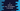# C program to calculate the sum of positive/negative numbers in an array### C program to calculate the positive and negative number sum from a user given integer array :

In this C programming tutorial, we will learn how to find the total sum of all positive numbers and all negative numbers in an integer array. The user will insert the elements into the array. To solve this problem, we will first ask the user to enter the total elements of the array. Then, we will take inputs for each position for that array. After the reading will be completed, we will scan the full array again and calculate the total sum of the positive and negative numbers. Let’s take a look at the program first :

### C program :

``````#include<stdio.h>

int main(){
//1
int total;
int i;

//2
int positiveSum = 0;
int negativeSum = 0;

//3
printf("How many numbers you want to add : ");
scanf("%d",&total);

//4
int numbers[total];

//5
for(i=0; i<total; i++){
printf("Enter number %d : ",(i+1));
scanf("%d",&numbers[i]);
}

//6
for(i=0 ; i<total ; i++){
if(numbers[i] < 0){
negativeSum += numbers[i];
}else{
positiveSum += numbers[i];
}
}

//7
printf("You have entered : \n");
for(i=0 ; i<total; i++){
printf("%d ",numbers[i]);
}

//8
printf("\nPositive numbers sum : %d",positiveSum);
printf("\nNegative numbers sum : %d\n",negativeSum);

}``````

### Explanation :

The commented numbers in the above program denote the step number below :

1. Create one integer variable total to store the total numbers and integer i to use in the loop below.
2. Create two integer variables to store the sum of all positive and negative numbers: positiveSum and negativeSum.
3. Ask the user to enter the total number of the array. Read and save it in total variable.
4. Create one integer array. The size of this array should be same as the total number entered by the user in the above step.
5. Run one for loop to read all numbers and store it in the array. Ask the user to enter each number, read it and store it to its corresponding position in the array.
6. After all, numbers are stored in the array, run one more loop to read each element of the array. Check each element: if it is a negative number, add it to the total sum of all negative numbers i.e. negativeSum. Similarly, if it is a positive number, add it to positiveSum.
7. At this position, negativeSum holds the total sum of all negative numbers and positiveSum holds the total sum of all positive numbers. Print all the numbers of the array that the user has entered.
8. Print out the sum of all positive numbers and sum of all negative numbers.

### Sample Output :

``````How many numbers you want to add : 4
Enter number 1 : 1
Enter number 2 : 2
Enter number 3 : -4
Enter number 4 : -5
You have entered :
1 2 -4 -5
Positive numbers sum : 3
Negative numbers sum : -9

How many numbers you want to add : 8
Enter number 1 : 1
Enter number 2 : 7
Enter number 3 : 8
Enter number 4 : -10
Enter number 5 : -9
Enter number 6 : 12
Enter number 7 : -5
Enter number 8 : 4
You have entered :
1 7 8 -10 -9 12 -5 4
Positive numbers sum : 32
Negative numbers sum : -24

How many numbers you want to add : 6
Enter number 1 : 1
Enter number 2 : 2
Enter number 3 : 3
Enter number 4 : -1
Enter number 5 : -2
Enter number 6 : -3
You have entered :
1 2 3 -1 -2 -3
Positive numbers sum : 6
Negative numbers sum : -6``````

You might also like :